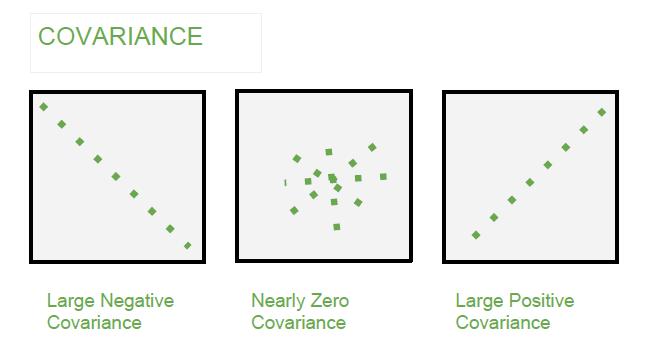/  Top Machine learning interview questions and answers   /  Explain in detail about Covariance?## Explain in detail about Covariance?

Covariance is a measure of how changes in one variable are associated with changes in a second variable. Precisely, covariance measures the degree to which two variables are linearly associated.

If the greater values of one variable mainly correspond with the greater values of the other variable, Lesser values of one variable corresponds to lesser values of other variable, then the covariance is positive.

Covariance can be calculated asWhere E(X) = mean of variable X

E(Y) = mean of variable Y